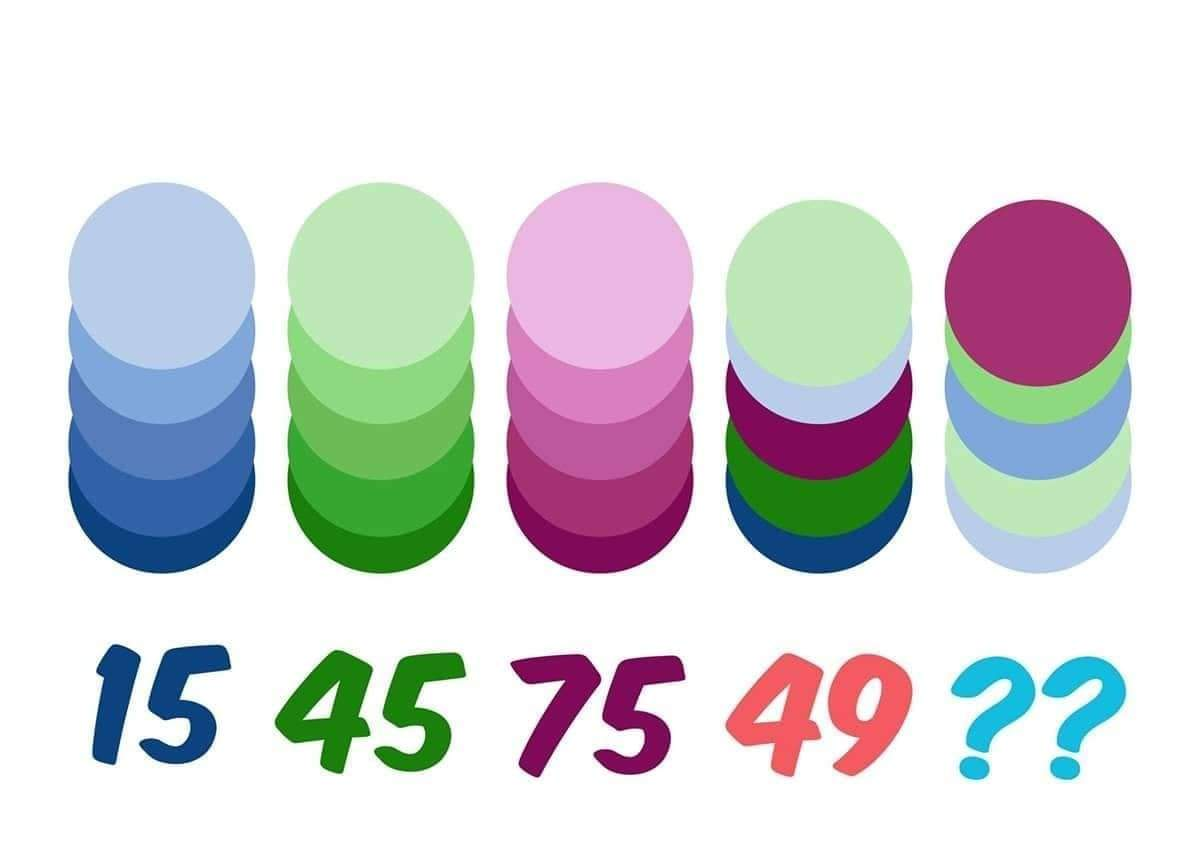# 15 45 75 49 What is the next number in the given picture

385.7K Views

In the given picture, First stack represents 15, next 45, next  75 and next 49 what will be the value of the next stack  ??Well matching the colors of the circles  and their correspondent value with the last figure of circles i think the answer is
14
Here is the thought process –
In first figure the values of each circle (from bottom to up)are =
X + X-5 + X-10 + X-15 + X-20 = 15
X is the darkest blue
So, x comes out to be 13
First row- 13,8,3,-2,-7
similarly the next figure values are = 19,14,9,4,-1
the values for the third figure = 25,20,15,10,5
the forth circle values (matching the colors of the circles with the previous three ) = 13,19,25,-7,-1
adding the values  of circles for each series we have the series as 15,45,75,49
so the fifth circle values according to their colors are = -7,-1,-2,4,20
so the total of this series of circles are = 14

And=32

1st column in ascending order values are 1-2-3-4-5, total 15

2nd column in ascending order is three times of first column. 3-6-9-12-15,total 45

3rd column in ascending order is five times of first column. 5-10-15-20-25, total 75

Hence value of every shaded circle is decided

4th column is 3+1+25+15+5, total49

Hence 5th column is 20+6+2+3+1=32